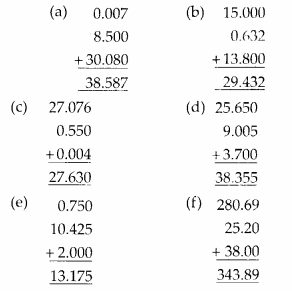# MP Board Class 6th Maths Solutions Chapter 8 Decimals Ex 8.5

## MP Board Class 6th Maths Solutions Chapter 8 Decimals Ex 8.5

Question 1.
Find the sum in each of the following :
(a) 0.007 + 8.5 + 30.08
(b) 15 + 0.632 + 13.8
(c) 27.076 + 0.55 + 0.004
(d) 25.65 + 9.005 + 3.7
(e) 0.75 + 10.425 + 2
(f) 280.69 + 25.2 + 38
Solution:Question 2.
Rashid spent Rs. 35.75 for Maths book and Rs. 32.60 for Science book. Find the total amount spent by Rashid.
Solution:
Money spent for Maths books = Rs. 35.75
Money spent for Science book = Rs. 32.60
Total money spent = Rs. 35.75 + Rs. 32.60 = Rs. 68.35
Therefore, total money spent by Rashid is Rs. 68.35

Question 3.
Radhika’s mother gave her Rs. 10.50 and her father gave her Rs. 15.80, find the total amount given to Radhika by the parents.
Solution:
Money given by mother = Rs. 10.50
Money given by father = Rs. 15.80
= Rs. 10.50 + Rs. 15.80 = Rs. 26.30Question 4.
Nasreen bought 3 m 20 cm cloth for her shirt and 2 m 5 cm cloth for her trouser. Find the total length of cloth bought by her.
Solution:
Cloth bought for shirt = 3 m 20 cm = 3.20 m
Cloth bought for trouser = 2 m 5 cm = 2.05 m
Total length of cloth bought by Nasreen
= 3.20 m + 2.05 m = 5.25 m
Therefore, total length of cloth bought by Nasreen is 5.25 m.

Question 5.
Naresh walked 2 km 35 m in the morning and 1 km 7 m in the evening. How much distance did he walk in all?
Solution:
Distance travelled in the morning
= 2 km 35 m = 2.035 km
Distance travelled in the evening
= 1 km 7 m = 1.007 km
Total distance travelled
= (2.035 + 1.007) km = 3.042 km
Therefore, total distance travelled by Naresh is 3.042 km.

Question 6.
Sunita travelled 15 km 268 m by bus, 7 km 7 m by car and 500 m on foot in order to reach her school. How far is her school from her residence?
Solution:
Distance travelled by bus
= 15 km 268 m = 15.268 km
Distance travelled by car
= 7 km 7 m = 7.007 km
Distance travelled on foot = 500 m = 0.500 km
Total distance travelled
= (15.268 + 7.007 + 0.500) km = 22.775 km
Therefore, total distance travelled by Sunita is 22.775 km.Question 7.
Ravi purchased 5 kg 400 g rice, 2 kg 20 g sugar and 10 kg 850g flour. Find the total weight of his purchases.
Solution:
Weight of Rice = 5 kg 400 g = 5.400 kg
Weight of Sugar = 2 kg 20 g = 2.020 kg
Weight of Flour = 10 kg 850 g = 10.850 kg
Total weight = (5.400 + 2.020 + 10.850) kg = 18.270 kg
Therefore, total weight of Ravi’s purchases is 18.270 kg.